# Investigation 2 – Lab### MINDSET

This Investigation is designed to:

• illustrate to you that work is force applied over a distance,
• allow you to discover that a simple machine makes work seem easier by changing the direction in which the effort force is applied,
• demonstrate to you that mechanical advantage is the ratio of load force to effort force,
• illustrate to you that mechanical advantage can also be measured as the ratio of effort arm length to load arm length,
• allow you to discover that increasing the number of pulleys in a system increases mechanical advantage by decreasing the amount of effort force needed to move the load, and
• allow you to discover that increasing the number of pulleys increases the distance over which a force is applied, thereby increasing mechanical advantage.

### BE PREPARED

Student Preparation for the Investigation includes having students gather the following materials. This preparation takes place on lab day after student lab groups have settled at their assigned lab tables.

Note: The materials are listed in students’ SDRs. They are also listed below for your reference.

• (1) fixed pulley
• (1) moveable pulley
• (3) 3m pieces of string
• (1) ring stand
• (1) pair of scissors
• (1) meter stick
• (1) metric ruler
• (3) 100-gram masses
• (1) white bucket
• (1) calculator
• (1) triple beam balance
• (1) spring scale (5N)

Have one student from your lab group collect the materials listed in your SDR.

### INVESTIGATE

• Reflect on the PreLab video as you move through the procedural steps.
• During the Experiment, every procedural step is important. If one step is skipped, data can become invalid. To help you keep on track, read each step thoroughly, complete the step, then check it off (Read it – Do it – Check it).
• Complete all of the procedural steps in your SDR.

Note: The procedural steps are listed below for your reference.

Trials 1-4:

1. In these Trials, you will investigate how changing the position of the load on a first-class lever affects the effort force required to lift the load.
1. How will moving the fulcrum of a first-class lever closer to the load affect the effort force needed to lift the load?
1. Determine the force of gravity on the load that will be used in the experiment. This is the load force.

A. Two 100 gram masses will be used as the load for the experiment. The masses that will be used are calibrated. Therefore, they do not need to be placed on a triple beam balance to determine their mass.

B. Calculate: Use the following formula to determine the force of gravity on the load. Remember to convert grams to kilograms. Round the answer to the nearest whole number.

Force of gravity (N) = mass (kg) x g     g = 9.8 m/s2

C. Record: Write the load force in each row of the column labeled “Load force” in Tables A and B on the last page of your Student Data Record.

4. Construct a first-class lever using the following steps: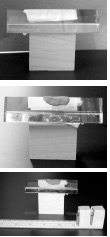A. Attach a wooden block to the prism using a piece of masking tape. This will be the fulcrum of the lever.

B. Place a small amount of clay over the fulcrum to help steady the ruler.

C. Attach two 100 gram masses to the 30 cm end of a metric ruler with two pieces of masking tape. This is the load.

D. Calibrate the spring scale. Hold it by the handle and move the adjustment nut until the indicator platform aligns with the “0” mark.

E. Record: Measure the force of gravity on the spring scale. Hold it upside down by the hook. Observe the force. Record the force above Table A.

F. Calibrate the spring scale for use in an upside-down position. Hold it by the metal hook. Turn the adjustment nut until the platform is even with the “0 N” mark on the Newton scale.

G. Hang the spring scale on the side of the ruler by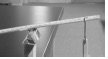placing the hook into the hole located at the 1.5 cm mark. If the ruler does not have a hole, use tape to secure the hook to the ruler at the 1.5 cm mark. This end of the ruler is the effort arm.

H. Place the lever system so that the end with the spring scale is off the table.

Trial 1:

1. Position the ruler so that the fulcrum is located at the 15 cm mark between the load and effort.
2. Determine the length of the load and effort arms.

A. Length of load arm = Distance from fulcrum to center of load. The center of the load is the point between the two 100 g masses.

B. Length of effort arm = Distance from the fulcrum to the center of the effort.

C. Record. Measure the length of the load arm and record the length in Table A.

D. Record. Measure the length of the effort arm and record the length in Table A.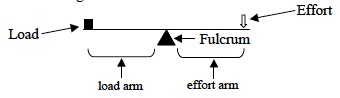1. Pull down on the spring scale until the ruler is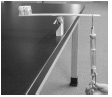completely horizontal.
2. Record: Look at the spring scale. Measure the force applied to the ruler when the ruler is completely horizontal. Read the amount of force applied to the ruler and add to it the force of the upside-down spring scale recorded above Table A. Write the sum in the column of Table A labeled “Effort Force (N)”. This is the effort force.

Trial 2:

1. Move the fulcrum to the 17 cm mark.
2. Repeat the procedures used in Trial 1 to measure the length of the effort arm, the length of the load arm, and the effort force.

Trial 3:

1. Move the fulcrum to the 19 cm mark.
2. Repeat procedures used in Trial 1 to measure the length of the effort arm, the length of the load arm, and the effort force.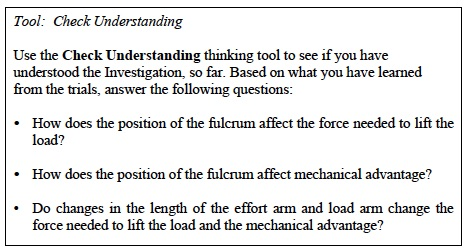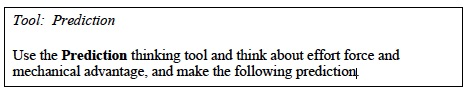• Predict what the effort force and mechanical advantage would be if the fulcrum were moved between the 0 cm and 15 cm mark on the ruler.

Trial 4:

1. Test your prediction. Move the fulcrum anywhere between the 0 cm and 15 cm mark on the ruler.

A. Record: Write the position of the fulcrum in Table A.

B. Record: Measure the length of the load arm and record the length in Table A.

C. Record: Measure the length of the effort arm and record the length in Table A.

D. Pull down on the spring scale until the ruler is completely horizontal.

E. Record: Look at the spring scale. Read the amount of force applied to the ruler and add to it the force of the upside-down spring scale recorded above Table A. Write the sum in the column of Table A labeled “Effort Force (N)”.

F. Calculate: Determine the mechanical advantage due to force and distance and record the results in Table A.

G. Compare the effort required to lift the load in this trial to Trial 1, 2, and 3. Did it feel easier or harder to lift the load in this trial? Why?Trials 5-8:

1. In these trials, you will investigate how changing the position of the load on a second class lever affects the effort force required to lift the load.
2. Begin to build a second class lever. Remove the prism and the woodblock from the first-class lever assembly.
3. Re-calibrate the spring scale for use in the normal upright position by holding the spring scale by the metal loop. Turn the adjustment nut until the platform is even with the “0 N” mark on the Newton scale. Refer to the Spring Scale (5N) Use and Operation procedure for assistance if necessary.
4. Construct a second-class lever as follows:

A. Remove the masses and masking tape from the end of the ruler.

B. Attach the two 100 gram masses to the ruler so that the two masses are touching each other at the 15 cm mark. This is the load.

C. The force of this load is 2 N, as measured in the first part of the experiment. This is already recorded in Table B in the Student Data Sheet.

Trial 5:

1. Construct the effort arm. Connect the spring scale to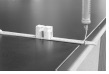the ruler by placing the hook end through the hole of the ruler at the 1.5 cm mark. If the ruler does not have a hole, use tape to secure the hook to the ruler at the 1.5 cm mark. This is the effort arm.
2. Find the length of the load and effort arms.

A. Length of load arm = Distance from fulcrum to center of load.

B. Length of effort arm = Distance from the fulcrum to the center of the effort.

C. Record: Measure the length of the load arm and record the length in Table B.

D. Record: Measure the length of the effort arm and record the length in Table B.1. Find the effort force required to lift the load 5 cm off the table.

A. Place the lever system so that the 4 cm mark is even with the table.

B. Hold the fulcrum end of the ruler against the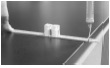table with the tips of your fingers. Use enough force to prevent the ruler from sliding when the effort force is applied.

C. Pull up on the spring scale until the spring scale is vertical. The platform should be at “0”.

D. Hold a metric ruler vertically beside the load. Pull up on the spring scale to lift the load until the edge of the lever is at the 5 cm mark.

E. Measure the force applied on the ruler. This is the effort force. Record in Table B.

Trial 6:

1. Move the load to the 11 cm mark.
2. Repeat the procedures from Trial 5 to measure the length of the effort arm, the length of the load arm, and the effort force.
3. Record your results in Table B.
4. Compare the effort required to lift the load in this trial to Trial 5. Did it feel easier or harder to lift the load in this trial?

Trial 7:

1. Move the load to the 7 cm mark.
2. Repeat the procedures from Trial 5 to measure the length of the effort arm, the length of the load arm, and the effort force.
3. Record your results in Table B.
4. Compare the effort required to lift the load in this trial to Trials 5 and 6. Did it feel easier or harder to lift the load in this trial?

Trial 8:

1. Move the load anywhere between the 15 cm and 30 cm mark on the ruler.

A. Where will the load be positioned?

B. Repeat the steps used in Trial 5 to determine the effort force needed to raise the load 5 cm off the table. Write the effort force in Table B.### CLEAN UP

Be sure to clean up your lab bench after completing the experiments.